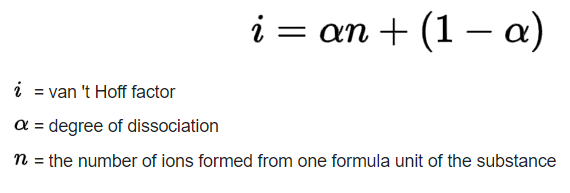# Ionization Factor Study Guide

INTRODUCTION

Jacobus Henricus van’t Hoff, Jr. was a Dutch chemist who explained why some chemical solutes undergo association or dissociation in a solution resulting in an abnormal molar mass and changes in colligative properties.

Typically, the molar mass of a solution is calculated by dividing its total mass by the moles of each substance dissolved within it. Sometimes, when we measure molar mass theoretically through the solution’s colligative properties, it gives a different value than when the value of molar mass is obtained experimentally.

This phenomenon is termed abnormal molar mass. The colligative properties of a solution depend on the ratio of solute molecules to solvent molecules and include osmotic pressure, freezing point depression, boiling point elevation, and relative lowering of vapor pressure.

### VAN’T HOFF FACTOR

The van’t Hoff Factor is used to measure the effect of solute molecules on the colligative properties of the solution. Colligative properties such as boiling point elevation, freezing point depression, osmotic pressure, and relative lowering of vapor pressure are proportional to the concentration of solute in the solution. We can find the van’t Hoff factor using the following equation:Source

We can predict a general value of the van’t Hoff constant of a solution based on whether it’s a nonelectrolyte or electrolyte.

Because nonelectrolytes dissolve but do not dissociate in water, the van’t Hoff constant for a nonelectrolyte solution is always 1. Let’s take a look at why this occurs using sucrose as an example. The chemical equation for the dissolution of sucrose in water is as follows:

sucrose (s) → sucrose (aq)

Here we see that a molecule of sucrose remains intact when dissolved, meaning that the solute particles do not dissociate into ions. Thus, α = and n = 0. If we plug this into the van’t Hoff equation, we find:

i = 1 + α(n – 1)

i = 1 + 0(0-1)

i = 1

Strong electrolytes dissociate completely when dissolved in water. Thus, their van’t Hoff constant is always greater than 1 and equal to the number of ions within the solution. Let’s use sodium chloride (NaCl) as an example. The chemical equation for the dissolution of sodium chloride in water is as follows:

NaCl (s) → Na+ (aq) + Cl– (aq);

Here, we can see that one NaCl molecule dissociates into one sodium ion and one chloride ion when dissolved in water. Thus, α = 1 and n = 2. When we plug this into the van’t Hoff equation, we find:

i = 1 + α(n – 1)

i = 1 + 0(0-1)

i = 1

i = 2

Sugar is a complex solute and does not dissociate when dissolved, so it won’t change the colligative properties of the solution. In the case of an ionic solute compound like salt, each molecule dissociates into two ions, and as a result the colligative properties of the solution do experience change.

Other forms of the van’t Hoff factor equation include:

i = observed colligative property/ theatrical colligative property

i = normal molar mass/ observed molar mass

i = an actual number of solute particles in the solution/ Expected number of solute particles in the solution.

## CONCLUSION

• Some solutes, like ionic solute compounds, undergo association or dissociation when they come in contact with the solvent.
• Association or dissociation of solute molecules results in a change of colligative properties of the solution and abnormal molar mass.
• The colligative properties of a solution are boiling point elevation, freezing point depression, osmotic pressure, and relative lowering of vapor pressure.
• Abnormal molar mass is the difference in molar mass obtained theoretically and the molar mass obtained experimentally.
• The van’t Hoff factor is denoted by the symbol ‘i’, which is also known as the van’t Hoff constant.
• The van’t Hoff factor is calculated by dividing the actual concentration of the particles produced by the concentration of solute by the concentration calculated from its molecular mass.

### FAQs

1. How do you find the ionization factor?

By dividing the actual concentration of the particles produced by the concentration of solute by the concentration calculated from its molecular mass.

2. What is the van’t Hoff factor? What is the significance of it?

van’t Hoff factor denotes the measurement of change in colligative properties of a solution when it comes in contact with a solute. It is used to explain the moral mass abnormality and change in colligative properties.

We hope you enjoyed studying this lesson and learned something cool about the Van’t Hoff Factor! Join our Discord community to get any questions you may have answered and to engage with other students just like you! Don’t forget to download our App to experience our fun VR classrooms – we promise, it makes studying much more fun! 😎

## SOURCES

1. Van’t Hoff Factor: https://www.vedantu.com/chemistry/vant-hoff-factor.Accessed 8th March 2022.
2. Van’t Hoff Factor Example: https://www.ck12.org/c/chemistry/electrolytes-and-colligative-properties/enrichment/Vant-Hoff-Factor-Example-1/. Accessed 8th March 2022.
]]>